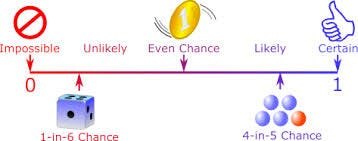# Basics of ProbabilityImage source mathsisfun.com

## What is probability?

As said by Walter Bagehot,

• Axiom 2: The probability that at least one of all the possible outcomes of a process (such as rolling a die) will occur is 1.
• Axiom 3: If two events A and B are mutually exclusive, then the probability of either A or B occurring is the probability of A occurring plus the probability of B occurring.

Artificial Intelligence at IISc